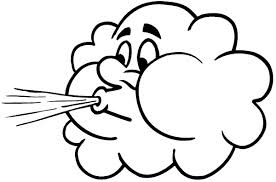Problems
256 - Wind Weight

Wind Weight

Time Limit: 1 sec

The Problem

The wind is X times heavier than water. The width, W length, L and height, H of a room in meter is given. Your task is to find how many kilos of air is there in the room.

Remember 1 cm3 water weight is 1 gm.The Input

The input file contains 4 floating numbers X(0 <= X <= 10), W(0 <= W <= 10000), L(0 <= L <= 10000, H(0 <= H <= 10000).

The Output

The output will print the weight of the wind in kilo rounded to 2 decimal points in  a separate line.

Sample Input

0.00129 16.00 12.00 4.00

Sample Output

990.72 kg

Problem Setter: Samia Safa Ahmed